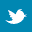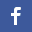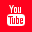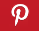Completely Solved C, C++ Programs Assignment.Quick Search Database Videos Tutorials Ebooks Forums FAQ Aboutus Household Industrial Manufacturing Service Shopping Transportation### C++ program to convert Rupee to Dollar or vice versa.

 Filed Under:

Problem Statement :
Design classes such that they support the following statements :-
Rupee r1,r2; Dollar d1,d2
d1 = r2(converts rupee to dollar)
r2 = d2(converts dollar to rupee)
Write a complete program which does such conversion according the world market values.

Logical Approach :
The above program is done using an Abstract Data Type (ADT) called class. A class is a mechanism to create objects. In this program two classes named Dollar and Rupee are created.

Class Dollar contains the following data member :
• dollar_val
and following member functions:
• Dollar()-Default constructor.
• Dollar(dollar)-Parameterized constructor, used to set a value in dollar.
• getDollar()-Used to get a dollar value.
• input_dollar()-Used to input a dollar value.

Class Rupee contains the following data member :
• rupee_val

and following member functions:
• Rupee ()-Default constructor.
• Rupee(rupee)-Parameterized constructor, used to set a value in rupee.
• getRupee()-Used to get a rupee value.
• input_rupee()-Used to input a dollar value.
• Rupee(Dollar)-Used to convert a dollar value to corresponding rupee value.
• operator Dollar()-Used to convert a rupee value to corresponding dollar value.

In this program we have used type conversion feature. Three types of situation might arise in the data conversion between incompatible types:
1. Basic to class type.
2. Class to basic type.
3. Class to class type.
Here we have done class to class conversion (Rupee to Dollar and vice-versa). A menu will appear on the screen from which user can choose which type of conversion he wants to do.

Algorithm :
Creating an ADT with name Dollar

Step 1:Creating an ADT Dollar

Step 2:Declaring private data members
double dollar_val//Declaring a double variable
Step 3:Declaring public member functions

&#61548; Dollar();
&#61548; Dollar(dollar);
&#61548; getDollar();
&#61548; input_dollar();

Default constructor(For Dollar class)

Dollar()
{
dollar_val=0.0;
}

Parameterized constructor(For Dollar class)

Dollar(dollar)
{
dollar_val=dollar;
}

This function is used to get a dollar value

getDollar()
{
return dollar_val;
}

This function is used to input a dollar value

input_dollar()
{
Read dollar_val;
}

Creating an ADT with name Rupee

Step 1:Creating an ADT Rupee

Step 2:Declaring private data members
double rupee_val//Declaring a double variable

Step 3:Declaring public member functions

• Rupee ();
• Rupee(rupee);
• getRupee();
• input_rupee();
• Rupee(Dollar);
• operator Dollar();

Default constructor(For Rupee class)

Rupee()
{
rupee_val=0.0;
}
Parameterized constructor(For Rupee class)

Rupee(double rupee)
{
rupee_val=rupee;
}

This function is used to get a rupee value

getRupee()
{
return rupee_val;
}

This function is used to input a rupee value

input_rupee()
{
Read rupee_val;
}

This function is used to convert a dollar value to corresponding rupee value

Rupee(Dollar d)
{
rupee_val=d.getDollar()*44.3203475;
}

This function is used to convert a rupee value to corresponding dollar value

operator Dollar()
{
return (Dollar(rupee_val/44.3203475));
}

Main procedure
while(true)
{
Print "Menu
1.Convert Dollar to Rupee
2.Convert Rupee to Dollar
3.Exit";
Read choice in ch;
If( ch=1)
{
Dollar d1;
Rupee r1;
d1.input_dollar();
r1=d1;
r1.getRupee();
}
Else if(ch=2)
{
Dollar d1;
Rupee r1;
r1.input_rupee();
d1=r1;
d1.getDollar();
}
Else if(ch= 3)
return 0;
}

Program listing :
/*C++ program to implement Conversion between INR and USD using data conversion*/

#include<iostream>

using namespace std;
//Creating an class with name Dollar
class Dollar
{
private:
double dollar_val;
public:
//Default constructor(For Dollar class)
Dollar()
{
dollar_val=0.0;
}
//Parameterized constructor(For Dollar class)
Dollar(double dollar)
{
dollar_val=dollar;
}
//This function is used to get a dollar value
double getDollar()
{
return dollar_val;
}
//This function is used to input a dollar value
void input_dollar()
{
cout<<"\nEnter an amount:";
cin>>dollar_val;
}
};
//Creating an class with name Rupee
class Rupee
{
private:
double rupee_val;
public:
//Default constructor(For Rupee class)
Rupee()
{
rupee_val=0.0;
}
//Parameterized constructor(For Rupee class)
Rupee(double rupee)
{
rupee_val=rupee;
}
//This function is used to get a Rupee value
double getRupee()
{
return rupee_val;
}
//This function is used to input a rupee value
void input_rupee()
{
cout<<"\nEnter an amount:";
cin>>rupee_val;
}
//This function is used to convert a dollar value to corresponding rupee value
Rupee(Dollar d)
{
rupee_val=d.getDollar()*44.3203475;
}
//This function is used to convert a rupee value to corresponding dollar value
operator Dollar()
{
return (Dollar(rupee_val/44.3203475));
}
};

int main()
{
int ch;
while(true)
{
cout<<"\nMenu\n-----------\n1.Convert Dollar to Rupee\n2.Convert Rupee to Dollar\n3.Exit\n";//Main menu
cout<<"Enter choice:";
cin>>ch;
switch(ch)
{
case 1:
{
Dollar d1;
Rupee r1;
d1.input_dollar();
r1=d1;
cout<<"\nEquivalent amount in Indian rupee:"<<r1.getRupee();
break;
}
case 2:
{
Dollar d1;
Rupee r1;
r1.input_rupee();
d1=r1;
cout<<"\nEquivalent amount in USA dollar:"<<d1.getDollar();
break;
}
case 3:
return 0;
default:
cout<<"Invalid choice\n";
}
}
return 0;
}

Output:
Menu
-----------
1.Convert Dollar to Rupee
2.Convert Rupee to Dollar
3.Exit
Enter choice:1

Enter an amount:16.50

Equivalent amount in Indian rupee:731.286
Menu
-----------
1.Convert Dollar to Rupee
2.Convert Rupee to Dollar
3.Exit
Enter choice:2

Enter an amount:990

Equivalent amount in USA dollar:22.3374
Menu
-----------
1.Convert Dollar to Rupee
2.Convert Rupee to Dollar
3.Exit
Enter choice:3

Discussions :
&#61548; Namespaces allow to group entities like classes, objects and functions under a name. This way the global scope can be divided in "sub-scopes", each one with its own name.

&#61548; All the files in the C++ standard library declare all of its entities within the std namespace. That is why we have generally included the using namespace std; statement in all programs that used any entity defined in iostream.

&#61548; Since the user defined are designed by us to suit our requirements, the compiler does not support automatic type conversions for such data types. We must, therefore, design the conversion routines by ourselves.

&#61548; We have done this program by using relation 1 U.S.D = 44.3203475 I.N.RBack to main directory:Software Practical

 @import url(http://www.google.com/cse/api/branding.css);